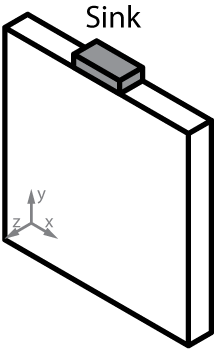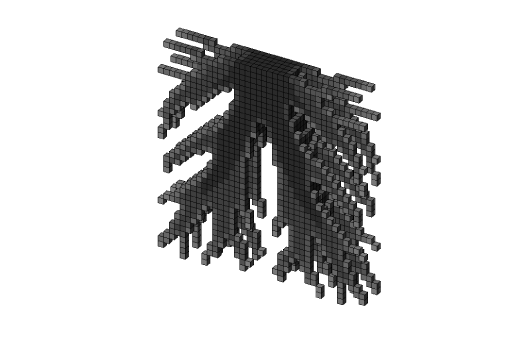# Heat conduction - Top3d

Heat in physics is defined as energy transferred between a system and its surrounding. The direct microscopic exchange of kinetic energy of particles through the boundary between two systems is called diffusion or heat conduction. In this tutorial, you will learn how to use top3d to solve heat conduction problems.

The implementation of heat conduction problems is not more complex than the one for compliant mechanism synthesis since the number of Degree Of Freedom (DOF) per node is one rather than three. Following the implementation of heat conduction problems in two dimensions, the implementation for three dimension problem as shown below is suggested in the following steps.### Step.1: Adding thermal conductivities

First, the elastic material properties (lines 8-10) are changed to the thermal conductivities of materials

k0   = 1;    % Good thermal conductivity
kmin = 1e-3; % Poor thermal conductivity


### Step.2: Changing Boundary conditions

The boundary conditions for the heat condition problem, i.e., a rectangular plate with a heat sink on the middle of top face and all nodes are given a thermal load as shown above, are changed corresponding (lines 10-18).

% USER-DEFINED SUPPORT FIXED DOFs
il = nelx/2-nelx/20:nelx/2+nelx/20; jl = nely; kl = 0:nelz;
fixedxy = il*(nely+1)+(nely+1-jl);
fixednid = repmat(fixedxy',size(kl)) + ...
repmat(kl*(nelx+1)*(nely+1),size(fixedxy,2),1);
fixeddof = reshape(fixednid,[],1);


### Step.3: Changing auxiliary variables

Since there is only one DOF per node in heat condition problems, some variables need to change correspondingly, such as $\texttt{ndof}$, $\texttt{edofMat}$.

Change the total number of DOFs and the force vector in lines 21-22

ndof = (nelx+1)*(nely+1)*(nelz+1);
F = sparse(1:ndof,1,-0.01,ndof,1);


The element conductivity matrix is called in line 25 by

KE = lk_H8(k0);


and it is defined in lines 99-145

function [KE] = lk_H8(k)
A1  = 4*eye(2);   A2 = -eye(2);
A3  = fliplr(A2); A4 = -ones(2);
KE1 = [A1 A2; A2 A1];
KE2 = [A3 A4; A4 A3];
KE  = 1/12 * k * [KE1 KE2; KE2 KE1];
end


The finite element connectivity matrix $\texttt{edofMat}$ is changed in lines 30-35

edofVec = nodeids(:)+1;
edofMat = repmat(edofVec,1,8)+ ...
repmat([0 nely + [1 0] -1 ...
(nely+1)*(nelx+1)+[0 nely + [1 0] -1]],nele,1);
iK = reshape(kron(edofMat,ones(8,1))',8*8*nele,1);
jK = reshape(kron(edofMat,ones(1,8))',8*8*nele,1);


### Step.4: Changing Finite Element Analysis Functions

The global conductivity matrix is assembled in a different way, hence line 70 need to change as

sK = reshape(KE(:)*(kmin+(1-kmin)*xPhys(:)'.^penal),8*8*nele,1);


The evulation of the objective function and analysis of the sensitivity are given in lines 75-76

c = sum(sum(sum((kmin+(1-kmin)*xPhys.^penal).*ce)));
dc = -penal*(1-kmin)*xPhys.^(penal-1).*ce;


### Step.5: Running program

The optimized topology is derived as shown below by promoting the following line in the Matlab:

top3d(40,40,5,0.30,3.0,1.4)